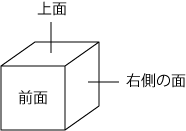Time Limit : sec, Memory Limit : KB

# Dice II

Construct a dice from a given sequence of integers in the same way as Dice I.

You are given integers on the top face and the front face after the dice was rolled in the same way as Dice I. Write a program to print an integer on the right side face.## Input

In the first line, six integers assigned to faces are given in ascending order of their corresponding labels. In the second line, the number of questions $q$ is given.

In the following $q$ lines, $q$ questions are given. Each question consists of two integers on the top face and the front face respectively.

## Output

For each question, print the integer on the right side face.

## Constraints

• $0 \leq$ the integer assigned to a face $\leq 100$
• The integers are all different
• $1 \leq q \leq 24$

## Sample Input

1 2 3 4 5 6
3
6 5
1 3
3 2


## Sample Output

3
5
6